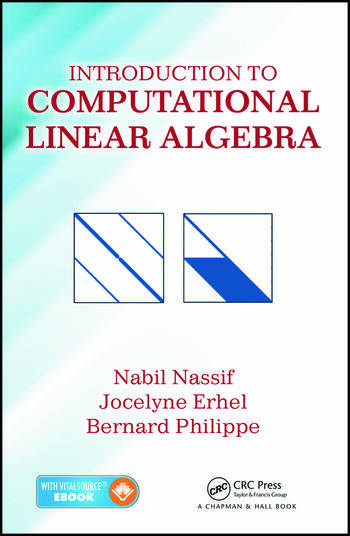# Introduction to Computational Linear Algebra

## 1st Edition

Nabil Nassif, Jocelyne Erhel, Bernard Philippe

Chapman and Hall/CRC
Published June 26, 2015
Textbook - 259 Pages - 9 B/W Illustrations
ISBN 9781482258691 - CAT# K24148

For Instructors Request Inspection Copy

was \$94.95

USD\$75.96

SAVE ~\$18.99

FREE Standard Shipping!

## Preview

### Summary

Teach Your Students Both the Mathematics of Numerical Methods and the Art of Computer Programming

Introduction to Computational Linear Algebra presents classroom-tested material on computational linear algebra and its application to numerical solutions of partial and ordinary differential equations. The book is designed for senior undergraduate students in mathematics and engineering as well as first-year graduate students in engineering and computational science.

The text first introduces BLAS operations of types 1, 2, and 3 adapted to a scientific computer environment, specifically MATLAB®. It next covers the basic mathematical tools needed in numerical linear algebra and discusses classical material on Gauss decompositions as well as LU and Cholesky’s factorizations of matrices. The text then shows how to solve linear least squares problems, provides a detailed numerical treatment of the algebraic eigenvalue problem, and discusses (indirect) iterative methods to solve a system of linear equations. The final chapter illustrates how to solve discretized sparse systems of linear equations. Each chapter ends with exercises and computer projects.

#### Instructors

We provide complimentary e-inspection copies of primary textbooks to instructors considering our books for course adoption.